Courses

# Dimensional And Model Analysis - 2

## 10 Questions MCQ Test Mock Test Series for Civil Engineering (CE) GATE 2020 | Dimensional And Model Analysis - 2

Description
This mock test of Dimensional And Model Analysis - 2 for GATE helps you for every GATE entrance exam. This contains 10 Multiple Choice Questions for GATE Dimensional And Model Analysis - 2 (mcq) to study with solutions a complete question bank. The solved questions answers in this Dimensional And Model Analysis - 2 quiz give you a good mix of easy questions and tough questions. GATE students definitely take this Dimensional And Model Analysis - 2 exercise for a better result in the exam. You can find other Dimensional And Model Analysis - 2 extra questions, long questions & short questions for GATE on EduRev as well by searching above.
QUESTION: 1

### A 1.0 m long model of a ship is tnwed in a towing tank at a speed of 81 cm/s. To what speed of the ship of 64 m long does this correspond?

Solution: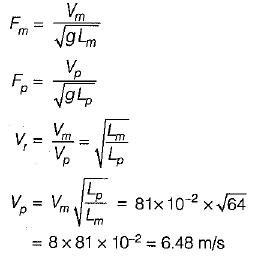QUESTION: 2

### A model boat, 1/100 size of its prototype has 0.12 N of resistance when simulating a speed of 5 m/s of the prototype. Water is the fluid in both cases. What is the corresponding resistance in the prototype? [The frictional forces can be neglected]

Solution:

The resistance offered at the free surface is the significant force and as such Froude model law is appropriate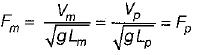If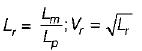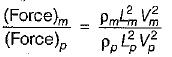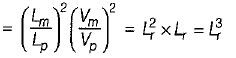∴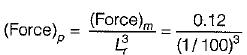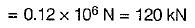QUESTION: 3

### Oil of density 917 kg/m3 and dynamic viscosity 0.29 Pa.s flows in a pipe of diameter 15 cm at a velocity of 2 m/s. What would be the velocity of flow of water in a 1 cm diameter pipe to make the two flows dynamically similar? The density and viscosity of water can be taken as 998 kg/m3 and 1.31 x 10-3 Pa.s respectivley.

Solution:

In this case, Reynolds similarity law is applicable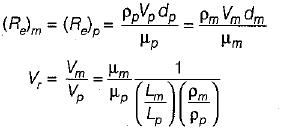Referring to oil with a subscript p and water with a suffix m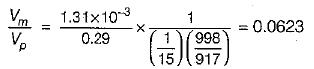∴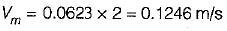QUESTION: 4

A 1 :5 scale model of a passenger car is tested in wind tunnel. The prototype velocity is 50 km/h. if the model drag is 200 N, what is the power required to overcome the drag in the prototype? The air in the model and prototype can be assumed to have the same properties.

Solution:

Reynolds similarity law is applicable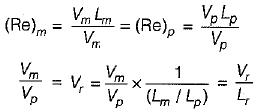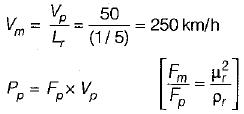∵ air is having same properties,
(Fm/Fp) - 1
∴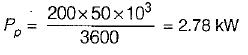QUESTION: 5

A pipe of diameter 1.5 m is required to transport an oil of relative density 0,9 and kinematic viscosity = 3 x 10-2 stoke at a rate of 3 m3/s. if a 15 cm diameter pipe with water at 20°C (n = 0.01 stoke) is used to model the above flow, the discharge in the model is

Solution:

The Reynolds number must be the same in the model and prototype for similar pipe flows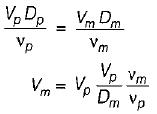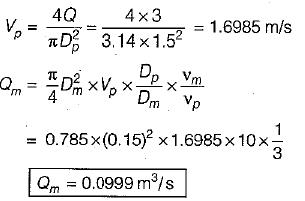QUESTION: 6

Both Reynolds number and Froude number assume, significance in one of the following example:

Solution:
QUESTION: 7

Match List-I (Parameter) with List-II (Scale ratio Froude Laws) the following: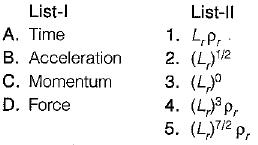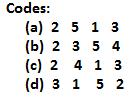Solution: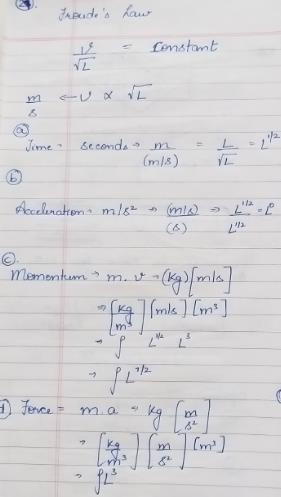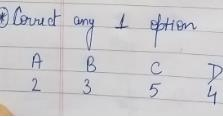QUESTION: 8

Match the List-I (Parameter) with List-ll (Scale ratios for Reynolds laws):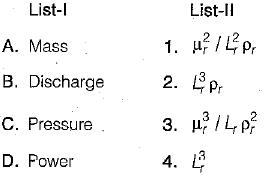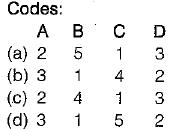Solution:
QUESTION: 9

Which one of the dimensionless numbers identifies the compressibility effect of a fluid

Solution:

At higher Mach number the compressibility effect is predominant.

QUESTION: 10

Geometric similarity between model and prototype means the similarity of

Solution:

Geometric similarity ⇒ Similarity in linear dimensions.